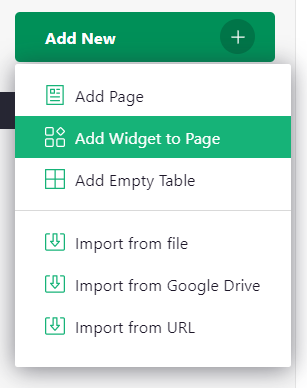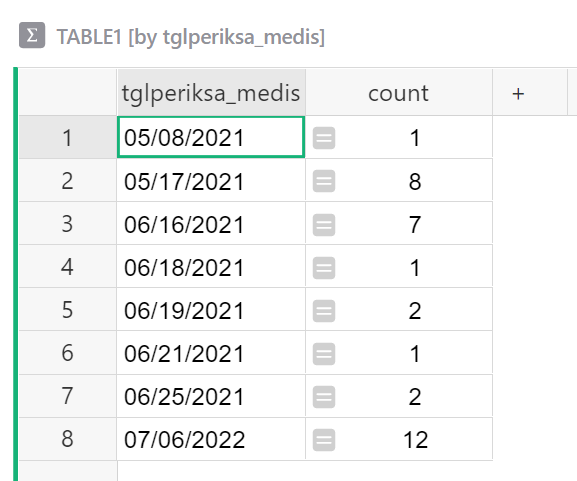# How to do traditional pivot table in grist

hi there,

I need help with my data, we use grist for transactions data, here our table:

I want to make calculation in column “produk_inv” just like traditional pivot table on excel/spreadsheet so we can get total count on every product, here that report we want to make:

is there any formula can work with this case it will be so good. thankyou

Hi there!

You can create a summary table of your data then add a few formula columns to get the data you need. With a summary table, data will update anytime a record is added to the initial table.

I created an example for you at the link below:

https://public.getgrist.com/knA4GGM31YhD/Community-1211/m/fork

In Table1, I recreated the table you provided above. Next, we want to create the summary table that you see at the bottom of the page.

To add a summary table, click on the green ‘Add New’ button then ‘Add Widget to Page’Under ‘Select Widget’, select ‘Table’. Under ‘Select Data’, select the table that contains your data. In the example, this is Table 1. Click the green Summation icon to create a summary table then under ‘Group By’, select tglperiksa_medis so we can group by date. Finally, click the green ‘Add to Page’ button.

Now, we have a summary table showing each date and the number of tests given each day.We’ll add more columns to get the data you want.

We’ll use len() and lookupRecords to find how many of a specific test was given on the specified date.

The formula in the ‘Swab Antigen’ column is:

``````len(Table1.lookupRecords(tglperiksa_medis=\$tglperiksa_medis, produk_inv="Swab Antigen"))
``````

`len()` counts the number of items in a list. We create a list of records using `lookupRecords`.

Our formula within the parenthesis looks up records in Table1 (our data table) where the date in Table1 matches the date in the summary table and where the value in the produk_inv column is `Swab Antigen`. A list of records is returned where both conditions are met. `len()` counts the number of records in that list.

The only thing that changes between the Swab Antigen column and PCR Test, Prevenar, etc. is the value within the double quotes at the end of the formula. We change which value we want to match to in the produk_inv column.

The formula for PCR Test is:

``````len(Table1.lookupRecords(tglperiksa_medis=\$tglperiksa_medis, produk_inv="PCR Test (PIMC)"))
``````

The formula for Prevenar is:

``````len(Table1.lookupRecords(tglperiksa_medis=\$tglperiksa_medis, produk_inv="Prevenar"))
``````

The formula for Telekonsultasi Pra-Vaksin is:

``````len(Table1.lookupRecords(tglperiksa_medis=\$tglperiksa_medis, produk_inv="Telekonsultasi Pra-Vaksin"))
``````

Let me know if you have any questions.

Thanks!
Natalie

1 Like